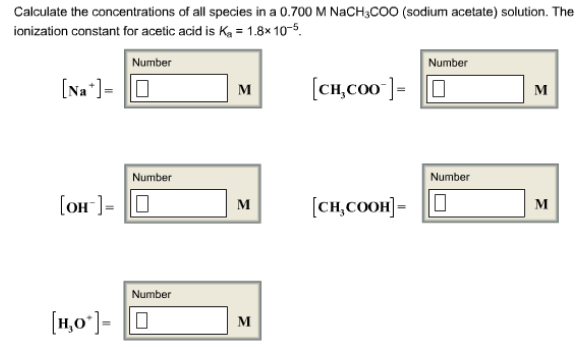# Problem: Calculate the concentrations of all species in a 0.700 M NaCH3COO (sodium acetate) solution. The ionization constant for acetic acid is K, = 1.8 x 10-5.[Na+] =                                                   [CH3COO-] = [OH-] =                                                   [CH3COOH] =[H3O+] =

🤓 Based on our data, we think this question is relevant for Professor Stenson's class at USOUTHAL.

###### FREE Expert Solution###### Problem Details

Calculate the concentrations of all species in a 0.700 M NaCH3COO (sodium acetate) solution. The ionization constant for acetic acid is K, = 1.8 x 10-5.

[Na+] =                                                   [CH3COO-] =

[OH-] =                                                   [CH3COOH] =

[H3O+] =